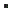AnUnt::GetBalance (Interface: AnUnt)Gets the balance of this Analytical Unit Account.DOUBLE GetBalance (    BSTR bsUnit,    BSTR bsKind)

Parameters
bsUnit
[in] The analytical unit of which you want to know the balance.
bsKind
[in] The analytical kind of which you want to know the balance.

Return value
A DOUBLE containing the balance of the given analytical unit.

RemarksIf bsUnit is left blank, then the balance for all analytical units is returned.If bsKind is left blank, then the balance for all analytical kinds is returned.The amount that is not distributed analytically is excluded from the returned balance.CreateAnUntGetBalanceC++ // Get the balance of the analytical unit with code = 'UNIT' combined with all analytical kinds double lfBalance = pAnUnt->GetBalance ("UNIT", ""); // Get the balance of the analytical unit with code = 'UNIT' combined with the analytical kind 'KIND' lfBalance = pAnUnt->GetBalance ("UNIT", "KIND"); // Get the balance of all analytical units combined with the analytical kind 'KIND' lfBalance = pAnUnt->GetBalance ("", "KIND"); // Get the balance of all analytical units combined with all analytical kinds lfBalance = pAnUnt->GetBalance ("", "");C# // Get the balance of the analytical unit with code = 'UNIT' combined with all analytical kinds double lfBalance = oAnUnt.GetBalance ("UNIT", ""); // Get the balance of the analytical unit with code = 'UNIT' combined with the analytical kind 'KIND' lfBalance = oAnUnt.GetBalance ("UNIT", "KIND"); // Get the balance of all analytical units combined with the analytical kind 'KIND' lfBalance = oAnUnt.GetBalance ("", "KIND"); // Get the balance of all analytical units combined with all analytical kinds lfBalance = oAnUnt.GetBalance ("", "");VBS Dim lfBalance ' Get the balance of the analytical unit with code = 'UNIT' combined with all analytical kinds lfBalance = oAnUnt.GetBalance("UNIT", "") ' Get the balance of the analytical unit with code = 'UNIT' combined with the analytical kind 'KIND' lfBalance = oAnUnt.GetBalance("UNIT", "KIND") ' Get the balance of all analytical units combined with the analytical kind 'KIND' lfBalance = oAnUnt.GetBalance("", "KIND") ' Get the balance of all analytical units combined with all analytical kinds lfBalance = oAnUnt.GetBalance("", "")VB.NET Dim lfBalance As Double ' Get the balance of the analytical unit with code = 'UNIT' combined with all analytical kinds lfBalance = oAnUnt.GetBalance("UNIT", "") ' Get the balance of the analytical unit with code = 'UNIT' combined with the analytical kind 'KIND' lfBalance = oAnUnt.GetBalance("UNIT", "KIND") ' Get the balance of all analytical units combined with the analytical kind 'KIND' lfBalance = oAnUnt.GetBalance("", "KIND") ' Get the balance of all analytical units combined with all analytical kinds lfBalance = oAnUnt.GetBalance("", "")